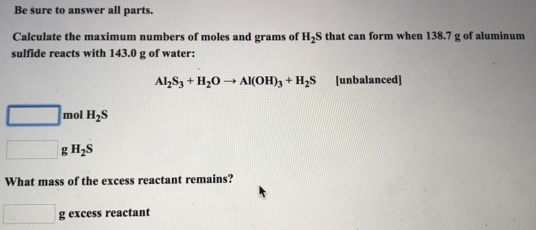# Problem: Be sure to answer all parts. Calculate the maximum numbers of moles and grams of H2S that can form when 138.7 g of aluminum sulfide reacts with 143.0 g of water: Al2S3 + H2O → Al(OH)3 + H2S [unbalanced] What mass of the excess reactant remains?

###### FREE Expert Solution
95% (441 ratings)###### Problem Details

Be sure to answer all parts.

Calculate the maximum numbers of moles and grams of H2S that can form when 138.7 g of aluminum sulfide reacts with 143.0 g of water:

Al2S3 + H2O → Al(OH)3 + H2S [unbalanced]

What mass of the excess reactant remains?